## Boxplots

In its simplest form, the boxplot presents five sample statistics - the minimum, the lower quartile, the median, the upper quartile and the maximum - in a visual display. The box of the plot is a rectangle which encloses the middle half of the sample, with an end at each quartile. The length of the box is thus the interquartile range of the sample. The other dimension of the box does not represent anything in particular. A line is drawn across the box at the sample median. Whiskers sprout from the two ends of the box until they reach the sample maximum and minimum. The crossbar at the far end of each whisker is optional and its length signifies nothing. The following diagram shows a dotplot of a sample of 20 observations (actual sample values used in the display) together with a boxplot of the same data.Although boxplots can be drawn in any orientation, most statistical packages seem to produce them vertically by default, as shown on the right, rather than horizontally. The length of the box becomes its height. The width across the page signifies nothing. Much more can be read from a boxplot than might be surmised from the simplistic method of its construction, particularly when the boxplots of several samples are lined up alongside one another (Parallel Boxplots). The box length gives an indication of the sample variability and the line across the box shows where the sample is centred. The position of the box in its whiskers and the position of the line in the box also tells us whether the sample is symmetric or skewed, either to the right or left. For a symmetric distribution, long whiskers, relative to the box length, can betray a heavy tailed population and short whiskers, a short tailed population. So, provided the number of points in the sample is not too small, the boxplot also gives us some idea of the "shape" of the sample, and by implication, the shape of the population from which it was drawn. This is all important when considering appropriate analyses of the data.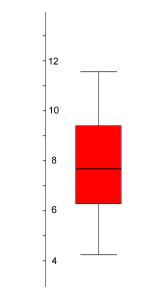### The Boxplot as an Indicator of Centrality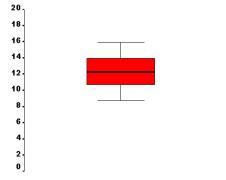The boxplot of a sample of 20 points from a population centred on 7. The boxplot of a sample of 20 points from a population centred on 12.

Quick Quiz

### The Boxplot as an Indicator of Spread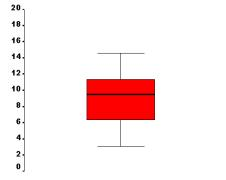The boxplot of a sample of 20 points from a population centred on 10 with standard deviation 1. The boxplot of a sample of 20 points from a population centred on 10 with standard deviation 3.

Quick Quiz

### The Boxplot as an Indicator of Symmetry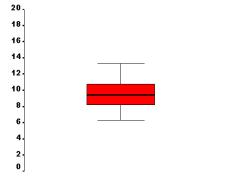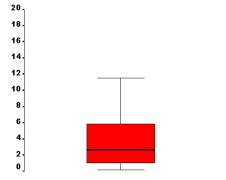The boxplot of a sample of 20 points from a symmetric population. The line is close to the centre of the box and the whisker lengths are the same. The boxplot of a sample of 20 points from a population which is skewed to the right. The top whisker is much longer than the bottom whisker and the line is gravitating towards the bottom of the box. The boxplot of a sample of 20 points from a population which is skewed to the left. The bottom whisker is much longer than the top whisker and the line is rising to the top of the box.

Quick Quiz

### The Boxplot as an Indicator of Tail Length

The tails are the extremities of the sample or population, rather than the centre. Lack of symmetry entails one tail being longer than the other. Populations are usually referred to as being heavy-tailed or light-tailed, or the Greek equivalent, leptokurtic (slender arched) or platykurtic (flat arched). The ideal level of kurtosis, neither too heavy or too light, is represented by the Normal population - the bell shaped curve. The box-plot of a sample from a Normal population should exhibit whiskers about the same length as the box, or perhaps marginally longer. The symmetric example above is from a Normal population.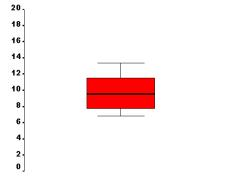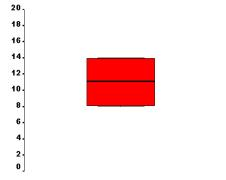The boxplot of a sample of 20 points from a population with long tails. The length of the whiskers far exceeds the length of the box. (A well proportioned tail would give rise to whiskers about the same length as the box, or maybe slightly longer.) The boxplot of a sample of 20 points from a population with short tails. The length of the whiskers is shorter than the length of the box. The boxplot of a sample of 20 points from a population with extremely short tails (actually a U-shaped population, with a dip in the middle rather than a hump). The whiskers are absent.

Quick Quiz

#### Note

Avoid making definitive statements about the shape (symmetry and kurtosis) of your boxplots when the size of your sample (i.e. the number of data points in it) is small (say, less than 10).

### Complications

The boxplots produced by statistical packages are rarely as described above. An attempt is made to alert you to sample values which may be unusually removed from the bulk of the data. These sample values are represented variously as circles or asterisks beyond the bounds of the whiskers. The whiskers thus do not extend to the minimum and maximum of the sample, but to the smallest and largest values inside a "reasonable" distance from the end of the box. This can considerably alter the whisker length of the plot. A highly skewed sample, for example, may appear to be reasonably symmetric in its box and whiskers with many values flagged as unusual beyond the whisker on one side. When interpreting these boxplots, it is a good idea to convert them to the simple form, by imagining the whiskers extend to the furthermost extreme points. The extra information provided by the flagging process enables you to distinguish between a truly skewed sample, and one whose apparent skewness is attributable to a single point at some remove from the rest of the data. Such a point or points may be an outlier; perhaps a measurement or data entry error, or a refugee from another population.

SPSS has a two stage flagging process. Values which are more than three box lengths from either end of the box receive an automatic red card. They are denoted by asterisks and entered in the referee's book as extreme values. Values which are between one and a half and three box lengths from either end of the box receive a yellow card and are entered in the book as outliers. You should bear in mind that the terms "outliers" and "extremes" carry connotations which the points may not deserve. The definitions of the yellow and red card zones are not entirely arbitrary, but not absolutely decreed either. They are to be interpreted as relative to a Normal population. If the population you are sampling from is not Normal, you may see many "outliers". The following diagram summarises an SPSS boxplot.Not so Quick Quiz

### Parallel Boxplots

The elegant simplicity of the boxplot makes it ideal as a means of comparing many samples at once, in a way that would be impossible for the histogram, say. Boxplots of the individual samples can be lined up side by side on a common scale and the various attributes of the samples compared at a glance. Obvious differences are immediately apparent. Data which will not lend itself to standard analysis can be identified. Large amounts of data can be made accessible.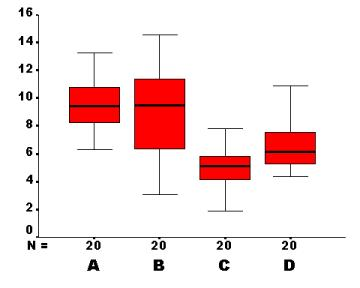In the above plot, sample A and B appear to have similar centres, which exceed those of C and D. Sample B appears to have larger variability than the other three samples. Samples A, B and C are reasonably symmetric, but sample D is skewed to the right. There are no obvious outliers in any of the samples.